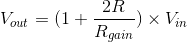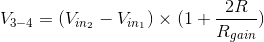# Instrumentation Amplifier

## Working principle

An instrumentation amplifier allows you to change its gain by varying one resistor value, Rgain, with the rest of the resistor values being equal (R), such that:### Formula derivation

Since any ideal op-amp tries to keep its two terminals at an equal voltage, connecting the negative terminals of the two op-amps to both sides of the Rgain resistor creates a voltage drop across Rgain equal to the voltage difference between Vin1 and Vin2. That voltage drop causes a current, which flows through the resistors (the feedback loops of the two op-amps draw no current). This produces a voltage drop across points 3 and 4 equal to:This voltage drop then gets amplified by the third amplifier by a gain of 1, assuming all resistor values other than Rgain are equal, yielding the first equation above.

This configuration allows to change the gain of the instrumentation amplifier by changing one resistance value, as well as have very high impedances connected to the positive terminals of the two amplifiers.

### Experiments

• Change the gain by varying the Rgain value.
• Increase the Rgain value in steps up to 10,000 Ohms, which simulates an infinite resistance – what value does the output voltage approach?